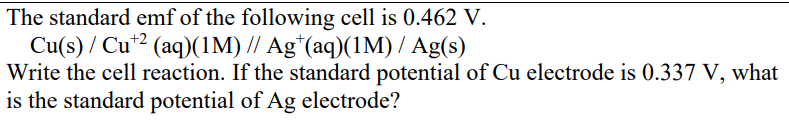# The standard emf of the following cell is 0.462 V. Cu(s) / Cu+2 (aq)(1M) // Ag+ (aq)(1M) / Ag(s) Write the cell reaction

more_vert

The standard emf of the following cell is 0.462 V. Cu(s) / Cu+2 (aq)(1M) // Ag+ (aq)(1M) / Ag(s) Write the cell reaction. If the standard potential of Cu electrode is 0.337 V, what is the standard potential of Ag electrode?